• 生活中经常使用的电梯是如何精确的把人们送到指定楼层的?机床又是如何做到精确切割物料的?伺服电机又是如何保证旋转位置精度的?这一切都要归功于一种神器——编码器，... 二、编码器分类  按照工作原理编码器可分为
• 本文主要讲了编码器分类及工作原理，下面一起来学习一下
• 生活中经常使用的电梯是如何精确的把人们送到指定楼层的?机床又是如何做到精确切割物料的?伺服电机又是如何保证旋转位置...这一切都要归功于一种神器——编码器，可是编码器又是什么?他又是如何精确的测量电机位置的呢?
• 使用LSTM自动编码器检测异常并对稀有事件进行分类。 如此多次，实际上是现实生活中的大多数数据，我们都有不平衡的数据。 数据是我们最感兴趣的事件，很少见，不像正常情况下那么频繁。 例如，在欺诈检测中。 ...
•研究论文
• ## 编码器分类：

千次阅读 2018-05-07 14:21:24
按工作原理：光电式、磁电式和触点电刷式 按码盘的刻孔方式：增量式和绝对式两类 由于博主接触面还不是很广，一共就用过两个种类的编码器，都是属于光电的 差分编码器:一般由8根线连接 信号线分别为 A+ A- B+ B- ...
按工作原理：光电式、磁电式和触点电刷式 按码盘的刻孔方式：增量式和绝对式两类 由于博主接触面还不是很广，一共就用过两个种类的编码器，都是属于光电的 差分编码器:一般由8根线连接 信号线分别为 A+ A- B+ B- Z+ Z- 以及VCC和GND 这里有一种不需要Z信号的，6线差分A+ A- B+ B- VCC 和GND 正交编码器：一般是5根线连接，信号线分别为A B Z VCC和GND
编码器线数: 就是旋转一圈你的A(B)会输出多少个脉冲 ,这里的A B就是上面的输出脉冲信号线，它们转一圈发出的脉冲数一样的,不过存在90°相位差 通常都是360线的 线数越高代表编码器能够反应的位置精度越高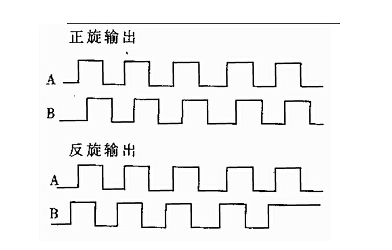相位差为90° 通过判断哪个信号在前 哪个信号在后 来决定TIM->COUNT是++ 还是 – 360线 AB一圈各为360个，Z信号为一圈一个
编码器信号： A 脉冲输出 B 脉冲输出 Z 零点信号 当编码器旋转到零点时，Z信号会发出一个脉冲表示现在是零位置 这个零点位置是固定，厂商指定的 VCC 电源通常分为24V的和5V的 GND 地线
这里需要注意： 1.这里的正交编码器是如果是24V的工作电压还需要用光耦隔离，24V转为3V3在接到STM32的定时器两个通道上 2.脉冲输出是OC门输出，需要上拉电阻 3.Z信号接到STM32的外部中断口上，很容易受到干扰 ，通常需要接一个电容到GND
这里给出一个24V转3.3V的隔离电路，用到的是6N136光耦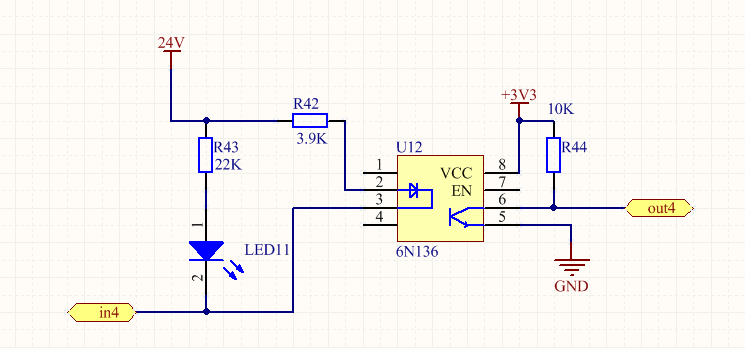硬件连接(这里使用的STM32F103ZET6的TIM4的CH1和CH2)： PB6–A PB7–B PA1–Z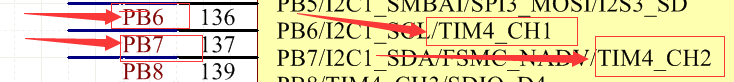代码详解： TIM4初始化代码如下:
#include "stm32f10x.h"
#include "encode.h"
#include "misc.h"
#include "nvic.h"
#include "sys.h"
#include "delay.h"

void TIM4_Mode_Config(void)
{
GPIO_InitTypeDef GPIO_InitStructure;
TIM_TimeBaseInitTypeDef  TIM_TimeBaseStructure;
TIM_ICInitTypeDef TIM_ICInitStructure;

//PB6 ch1  A,PB7 ch2
RCC_APB1PeriphClockCmd(RCC_APB1Periph_TIM4, ENABLE);//使能TIM4时钟
RCC_APB2PeriphClockCmd(RCC_APB2Periph_GPIOB, ENABLE);//使能GPIOA时钟

GPIO_StructInit(&GPIO_InitStructure);//将GPIO_InitStruct中的参数按缺省值输入
GPIO_InitStructure.GPIO_Pin = GPIO_Pin_6 | GPIO_Pin_7;
GPIO_InitStructure.GPIO_Mode = GPIO_Mode_IN_FLOATING;//PA6 PA7浮空输入
GPIO_InitStructure.GPIO_Speed = GPIO_Speed_50MHz;
GPIO_Init(GPIOB, &GPIO_InitStructure);

NVIC_Config(2);

TIM_DeInit(TIM4);
TIM_TimeBaseStructInit(&TIM_TimeBaseStructure);
TIM_TimeBaseStructure.TIM_Period = 359*4;  //设定计数器重装值   TIMx_ARR = 359*4
TIM_TimeBaseStructure.TIM_Prescaler = 0; //TIM3时钟预分频值
TIM_TimeBaseStructure.TIM_ClockDivision =TIM_CKD_DIV1 ;//设置时钟分割 T_dts = T_ck_int
TIM_TimeBaseStructure.TIM_CounterMode = TIM_CounterMode_Up; //TIM向上计数
TIM_TimeBaseInit(TIM4, &TIM_TimeBaseStructure);

TIM_EncoderInterfaceConfig(TIM4, TIM_EncoderMode_TI12, TIM_ICPolarity_BothEdge ,TIM_ICPolarity_BothEdge);//使用编码器模式3，上升下降都计数
TIM_ICStructInit(&TIM_ICInitStructure);//将结构体中的内容缺省输入
TIM_ICInitStructure.TIM_ICFilter = 6;  //选择输入比较滤波器
TIM_ICInit(TIM4, &TIM_ICInitStructure);//将TIM_ICInitStructure中的指定参数初始化TIM3

TIM_ClearFlag(TIM4, TIM_FLAG_Update);//清除TIM3的更新标志位
TIM_ITConfig(TIM4, TIM_IT_Update, ENABLE);//运行更新中断
//Reset counter
TIM4->CNT = 0;//

TIM_Cmd(TIM4, ENABLE);   //启动TIM4定时器

}

void TIM4_Init(void)
{
TIM4_Mode_Config();
}
这里的NVIC_Config(2)是我个人写的一种多种中断配置的方法单独放在nvic.c中需要了解的可以自己看看工程
这里通常要问的是两点 1.TIM_TimeBaseStructure.TIM_Period = 359*4 2.TIM_EncoderInterfaceConfig(TIM4, TIM_EncoderMode_TI12, TIM_ICPolarity_BothEdge ,TIM_ICPolarity_BothEdge);//使用编码器模式3，上升下降都计数
很多人不理解为要360线的编码器为什么这里的重装值乘以4 读出来的为什么又要/4，其实这两个要结合起来解释 首先看看这个函数TIM_EncoderInterfaceConfig,它有4个参数 1.选择哪个定时器 即TIM4 2.编码器模式有三种 见下图 3.TIM_IC1的极性 4.TIM_IC2的极性 这里设置的是编码器模式3，且TI1和TI2都是双边沿触发–即上下边沿都计数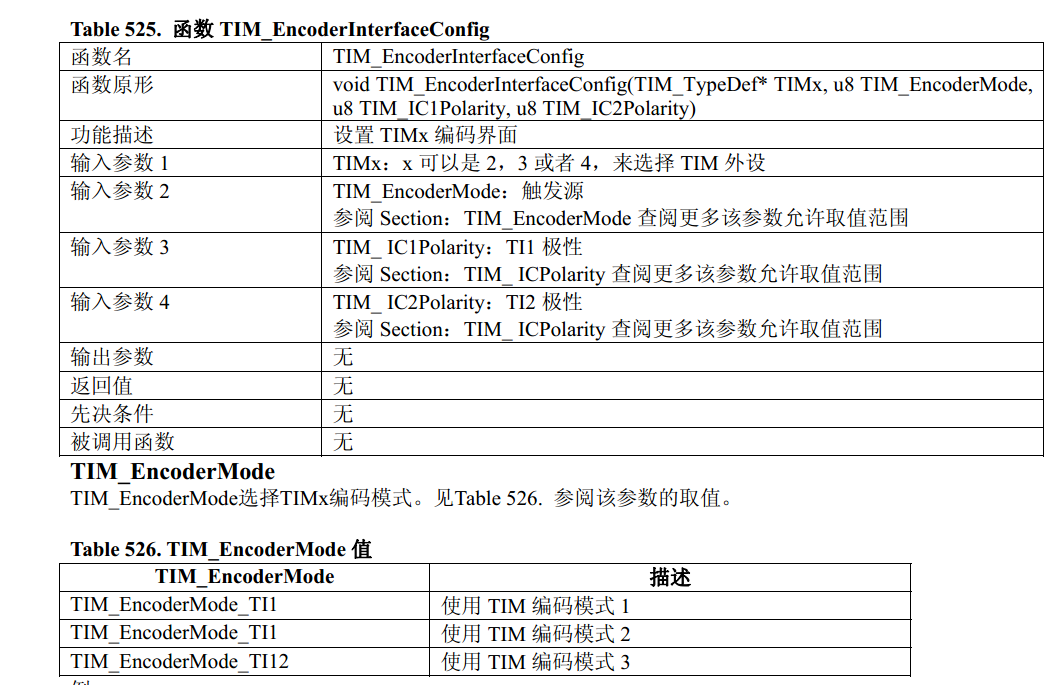还有一个大家不是很懂的图，我来分析一下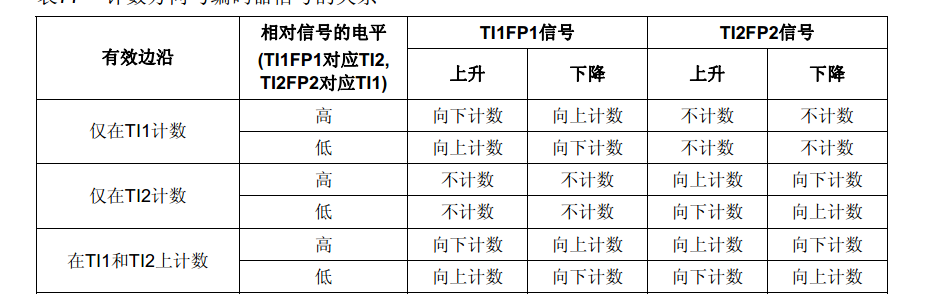1.有效边沿 其实就是对应上面设置的编码器的三种模式 2.相对信号的电平,这里没有理解手册意思，我把它理解为于它的高低电平意味着将PB6和PB7接口对换，PB7接A PB6接B 这样一来就意味着原来的正转变成反转 计数上升变为下降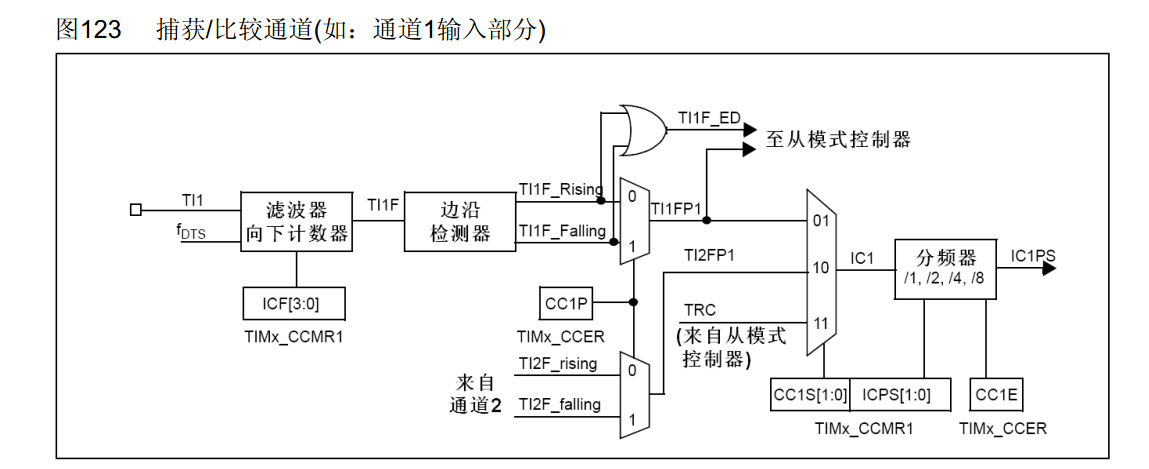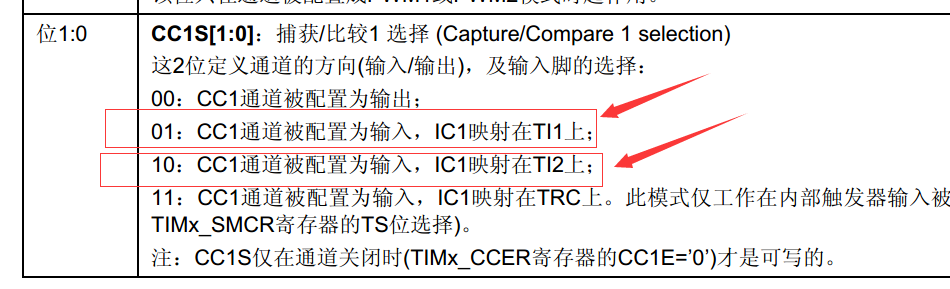TIx 就相当于输入信号的 TIM4->CH1 TIM4->CH2 TIxF 滤波后信号 TIxFPx经过带极性选择的边缘检测器过后的产生的信号
3.至于TI1FP1和TI2FP2信号在上身沿计数还是下降沿计数受两点影响 极性(是否反向) 边缘检测(上升沿还是下降沿) 我们这里设置的是不反向 在双边沿计数,即在A上升下降 B的上身下降都计数
而计数为什么是x4倍 ，下图结合上面的配置详细说明了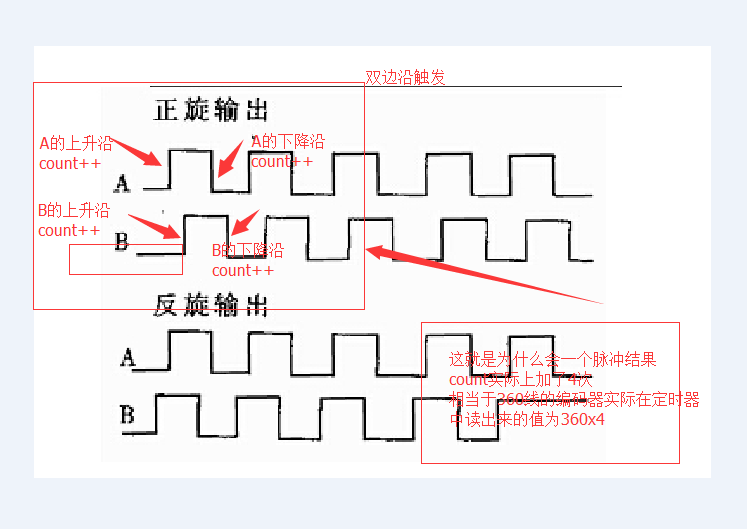由此完成了编码器的配置
至于读取编码器角度的时间，要根据实际需要来设置
编码器线数为 w线/圈 转速为 V 圈/min 读取间隔时间 t(线间隔时间)
t <= 60/WV 单位为秒
还有Z信号归零，在遇到Z信号的时候，将定时器的CNT=0，这样就能保证位置与CNT实际对应上了 中断代码如下
//外部中断1，编码器Z相归零  优先级--①  0 0
void EXTI1_IRQHandler(void)
{

TIM4->CNT = 0;//每次遇到相对零(Z信号)就将计数归0
TIM_Cmd(TIM4, ENABLE);
EXTI_ClearITPendingBit(EXTI_Line1);

}

//编码器接口模式     优先级--2   1  1
void TIM4_IRQHandler(void)
{
if(TIM4->SR&0x0001)//溢出中断
{
;
}
TIM4->SR&=~(1<<0);//清除中断标志位
}
先看手册 
展开全文• 问题描述：老师让用自编码器做一个多分类的问题，但是自编码器是无监督学习，那么在提取特征之后怎么分类呢？ 像CNN的话，就可以直接把标签当最后一层的输出去分类，但是自编码器输入的数据应该是没有标签的，而且...机器学习 深度学习 神经网络
• MATLAB给出了堆栈自编码器的实现Train Stacked Autoencoders for Image Classification ，本文对其进行分析 堆栈自编码器Stacked Autoencoders 堆栈自编码器是 具有多个隐藏层的神经网络可用于解决图像等复杂数据的...
在前面两篇博客的基础上，可以实现单层自编码器网络（一个解码器+一个解码器）。对于多层自编码器的实现，MATLAB给出了堆栈自编码器用于图像分类的网页Train Stacked Autoencoders for Image Classification ，本文对其进行翻译和学习。
堆栈自编码器Stacked Autoencoders
具有多个隐藏层的神经网络可用于解决图像等复杂数据的分类问题。每个层都可以在不同的抽象级别学习特性。然而，训练具有多个隐藏层的神经网络在实践中可能是困难的。一种有效训练多层神经网络的方法是一次训练一层。可以通过为每个隐藏层训练一种称为自编码器的特殊网络来实现这一点。
下面的例子展示了如何训练具有两个隐藏层的神经网络来对数字图像进行分类。首先，使用自编码器以无监督的方式单独训练隐藏层。然后训练最后一个softmax层，并将这些层连接在一起，形成一个堆叠的网络，在有监督的情况下对其进行最后一次训练。
代码实现
代码较长，因此注释也比较多，但是其基本思想并不复杂，实现的过程也容易理解。
%% Train Stacked Autoencoders for Image Classification训练用于图像分类的堆栈自编码器
%% 导入训练集
% 此示例始终使用合成数据进行训练和测试。已通过对使用不同字体创建的数字图像应用随机仿射变换来生成合成图像
% 每个数字图像为 28×28 像素，共有 5000 个训练样本。您可以加载训练数据，并查看其中一些图像
% Load the training data into memory 加载自带的手写数字数据集
clear;
clc;
[xTrainImages,tTrain] = digitTrainCellArrayData;
% 展示图像
clf
for i = 1:20
subplot(4,5,i);
imshow(xTrainImages{i});
end
%% Training the first autoencoder 训练第一个自编码器
% 首先在不使用标签的情况下基于训练数据训练稀疏自编码器。
% 自编码器是一种神经网络，该网络会尝试在其输出端复制其输入。因此，其输入的大小将与其输出的大小相同。
% 当隐含层中的神经元数量小于输入的大小时，自编码器将学习输入的压缩表示。
% 神经网络在训练前具有随机初始化的权重。因此，每次训练的结果都不同。为避免此行为，请显式设置随机数生成器种子。
rng('default')%确保随机数
hiddenSize1 = 100;%设置自编码器的隐含层的大小。对于要训练的自编码器，最好使隐含层的大小小于输入大小。
% 训练设置
autoenc1 = trainAutoencoder(xTrainImages,hiddenSize1, ...
'MaxEpochs',400, ...    % 最大训练轮次400个
'L2WeightRegularization',0.004, ...   % L2正则项对网络权重的影响，通常非常小
'SparsityRegularization',4, ...       % 稀疏正则项的系数
'SparsityProportion',0.15, ...        % 稀疏比例
'ScaleData', false);     %输入数据不进行缩放
% 查看第一个自编码器的结构
view(autoenc1)
% 可视化第一个自编码器的权重
% 自编码器的编码器部分所学习的映射可用于从数据中提取特征。
% 编码器中的每个神经元都具有一个与之相关联的权重向量，该向量将进行相应调整以响应特定可视化特征。您可以查看这些特征的表示。
figure()
plotWeights(autoenc1);
% 可以看到，自编码器学习的特征代表了数字图像中的弯曲和笔划图案。
% 自编码器的隐含层的 100 维输出是输入的压缩版本，它汇总了对上面可视化特征的响应。
% 基于从训练数据中提取的一组向量训练下一个自编码器。首先，必须使用经过训练的自编码器中的编码器生成特征。
feat1 = encode(autoenc1,xTrainImages); % 自编码器1的输出
%% Training the second autoencoder  训练第二个自编码器
% 以相似的方式训练第二个自编码器，主要区别在于使用第一个自编码器的输出作为第二个自编码器的输入。
% 还需要将隐含表示的大小减小到 50，以便第二个自编码器中的编码器学习输入数据的更小表示。
hiddenSize2 = 50;
autoenc2 = trainAutoencoder(feat1,hiddenSize2, ...
'MaxEpochs',100, ...
'L2WeightRegularization',0.002, ...
'SparsityRegularization',4, ...
'SparsityProportion',0.1, ...
'ScaleData', false);
% 查看第二个自编码器的结构
view(autoenc2)
% 自编码器2的输出
feat2 = encode(autoenc2,feat1);
%% Training the final softmax layer 训练最后的分类层softmax
% 训练数据中的原始向量具有 784 个维度。原始数据通过第一个编码器后，维度减小到 100 维。
% 应用第二个编码器后，维度进一步减小到 50 维。
% 训练最后一层softmax层以对 50 维特征向量进行分类。
% 与自编码器不同，这里使用训练数据的标签以有监督方式训练 softmax 层。
softnet = trainSoftmaxLayer(feat2,tTrain,'MaxEpochs',400);%trainSoftmaxLayer(输入特征，输出标签，参数(循环次数)，参数值)
% 显示softmax网络
view(softnet)
% 将自编码器中的编码器与 softmax 层堆叠在一起，以形成用于分类的堆叠网络
deepnet = stack(autoenc1,autoenc2,softnet);%网络合成
view(deepnet) % 深度网络的显示
%% 构建测试集并进行测试
% 在搭建了完整网络之后，可以基于测试集计算结果。要将图像用于堆叠网络，必须将测试图像重构为矩阵。
% 这可以通过先堆叠图像的各列以形成向量，然后根据这些向量形成矩阵来完成。
% 获取每个图像中的像素数
imageWidth = 28;
imageHeight = 28;
inputSize = imageWidth*imageHeight; % 输入矩阵的大小
% 载入测试图像
[xTestImages,tTest] = digitTestCellArrayData;
% 图像转换为向量并放在矩阵中
xTest = zeros(inputSize,numel(xTestImages)); %生成一个0矩阵，numel计算图像中的元素个数
for i = 1:numel(xTestImages)
xTest(:,i) = xTestImages{i}(:);
end
% 网络测试
y = deepnet(xTest); %测试结果输出
plotconfusion(tTest,y); % 测试结果对比，使用混淆矩阵来可视化结果
%% Fine tuning the deep neural network 微调深度神经网络
% 通过对整个多层网络执行反向传播，可以改进堆叠神经网络的结果，此过程通常称为微调。
% 通过以有监督方式基于训练数据重新训练网络来进行微调。在执行此操作之前，必须将训练图像重构为矩阵，就像对测试图像所做的那样。
xTrain = zeros(inputSize,numel(xTrainImages));
for i = 1:numel(xTrainImages)
xTrain(:,i) = xTrainImages{i}(:);
end
% 进行微调
deepnet = train(deepnet,xTrain,tTrain);
%再次显示结果并对比
y = deepnet(xTest);
plotconfusion(tTest,y);


展开全文神经网络 深度学习 matlab
• 主要介绍了keras自动编码器实现系列之卷积自动编码器操作，具有很好的参考价值，希望对大家有所帮助。一起跟随小编过来看看吧
• 我们使用异常检测的概念为这些过程构建了一个自动编码器分类器。 但是，我们拥有的数据是一个时间序列。但是之前我们使用了Dense层自动编码器，它不使用数据中的时间特征。因此，在这篇文章中，我们将通过构建LSTM...
在这里，我们将学习LSTM模型的数据准备细节，并构建用于稀有事件分类的LSTM Autoencoder。
这篇文章是我之前使用Autoencoders发布极端罕见事件分类的延续。在上一篇文章中，我们讨论了极少数事件数据中的挑战，其中正面标记数据少于1％。我们使用异常检测的概念为这些过程构建了一个自动编码器分类器。
但是，我们拥有的数据是一个时间序列。但是之前我们使用了Dense层自动编码器，它不使用数据中的时间特征。因此，在这篇文章中，我们将通过构建LSTM Autoencoder来改进我们的方法。
在这里，我们将学习：
LSTM模型的数据准备步骤， 构建和实现LSTM自动编码器，以及 使用LSTM自动编码器进行罕见事件分类。 快速回顾LSTM：
LSTM是一种递归神经网络（RNN）。一般而言，RNN和LSTM特别用于顺序或时间序列数据。 这些模型能够自动提取过去事件的影响。 LSTM因其能够提取过去事件的长期和短期影响而闻名。 在下文中，我们将直接开发LSTM Autoencoder。关于数据问题，我们有关于纸张制造的纸张断裂的实际数据。我们的目标是提前预测休息时间。有关数据，问题和分类方法的详细信息，请参阅使用自动编码器的极端罕见事件分类。
LSTM多变量数据自动编码器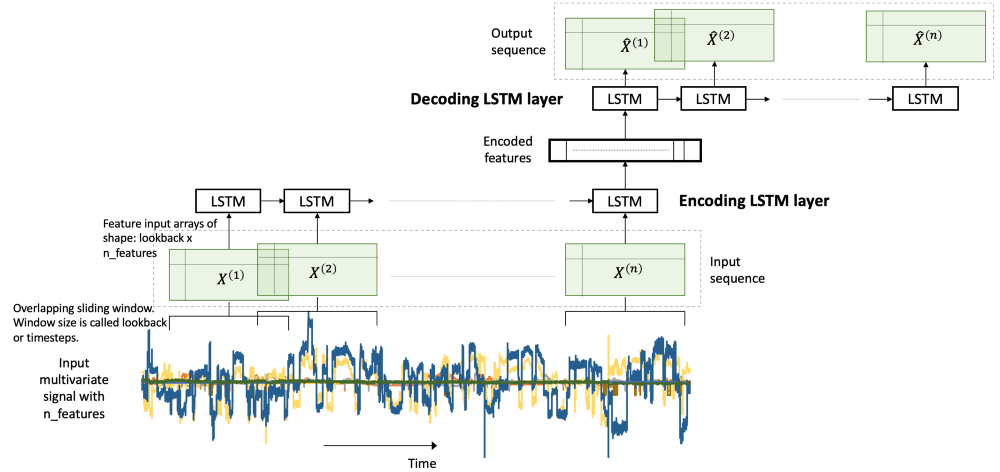在我们的问题中，我们有一个多变量的时间序列数据。 多变量时间序列数据包含在一段时间内观察到的多个变量。 我们将在此多变量时间序列上构建LSTM自动编码器，以执行罕见事件分类。 如中所述，这是通过使用异常检测方法实现的：
我们在正常（负标记）数据上构建自动编码器， 用它来重建一个新的样本， 如果重建错误很高，我们将其标记为换页符。 LSTM几乎不需要特殊的数据预处理步骤。 在下文中，我们将充分注意这些步骤。
让我们来实现。
Libraries
I like to put together the libraries and global constants first.
%matplotlib inline
import matplotlib.pyplot as plt
import seaborn as sns

import pandas as pd
import numpy as np
from pylab import rcParams

import tensorflow as tf
from keras import optimizers, Sequential
from keras.models import Model
from keras.utils import plot_model
from keras.layers import Dense, LSTM, RepeatVector, TimeDistributed
from keras.callbacks import ModelCheckpoint, TensorBoard

from sklearn.preprocessing import StandardScaler
from sklearn.model_selection import train_test_split
from sklearn.metrics import confusion_matrix, precision_recall_curve
from sklearn.metrics import recall_score, classification_report, auc, roc_curve
from sklearn.metrics import precision_recall_fscore_support, f1_score

from numpy.random import seed
seed(1)
from tensorflow import set_random_seed
set_random_seed(123)
from sklearn.model_selection import train_test_split

SEED = 123 #used to help randomly select the data points
DATA_SPLIT_PCT = 0.2

rcParams['figure.figsize'] = 8, 6
LABELS = ["Normal","Break"]

数据准备
如前所述，LSTM需要在数据准备中采取一些具体步骤。 LSTM的输入是根据时间序列数据创建的三维阵列。 这是一个容易出错的步骤，因此我们将查看详细信息。
Read data The data is taken from . The link to the data is here.
df = pd.read_csv("data/processminer-rare-event-mts - data.csv")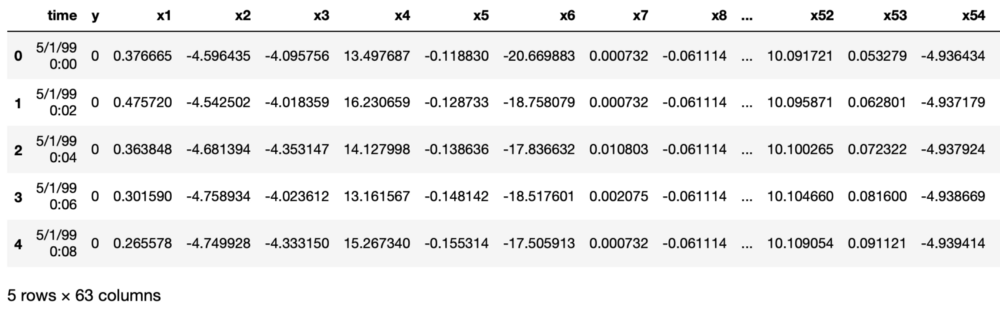Curve Shifting
正如中所提到的，这种罕见事件的目的是在片断发生之前预测片断。 我们将尝试提前预测4分钟。 对于此数据，这相当于将标签向上移动两行。 可以使用df.y = df.y.shift（-2）直接完成。 但是，这里我们要求执行以下操作，
对于带有标签1的任何行n，make（n-2）? n-1）为1.这样，我们教导分类器预测最多4分钟。 和， 删除行n。 行n被删除，因为我们不想教分类器来预测已经发生的中断。 我们开发了以下功能来执行此曲线移位。
sign = lambda x: (1, -1)[x < 0]

def curve_shift(df, shift_by):
'''
This function will shift the binary labels in a dataframe.
The curve shift will be with respect to the 1s.
For example, if shift is -2, the following process
will happen: if row n is labeled as 1, then
- Make row (n+shift_by):(n+shift_by-1) = 1.
- Remove row n.
i.e. the labels will be shifted up to 2 rows up.

Inputs:
df       A pandas dataframe with a binary labeled column.
This labeled column should be named as 'y'.
shift_by An integer denoting the number of rows to shift.

Output
df       A dataframe with the binary labels shifted by shift.
'''

vector = df['y'].copy()
for s in range(abs(shift_by)):
tmp = vector.shift(sign(shift_by))
tmp = tmp.fillna(0)
vector += tmp
labelcol = 'y'
# Add vector to the df
df.insert(loc=0, column=labelcol+'tmp', value=vector)
# Remove the rows with labelcol == 1.
df = df.drop(df[df[labelcol] == 1].index)
# Drop labelcol and rename the tmp col as labelcol
df = df.drop(labelcol, axis=1)
df = df.rename(columns={labelcol+'tmp': labelcol})
# Make the labelcol binary
df.loc[df[labelcol] > 0, labelcol] = 1

return df

我们现在将移动数据并验证移位是否正确。 在随后的部分中，我们还有更多的测试步骤。 建议使用它们以确保数据准备步骤按预期工作。
print('Before shifting')  # Positive labeled rows before shifting.
one_indexes = df.index[df['y'] == 1]
display(df.iloc[(np.where(np.array(input_y) == 1)-5):(np.where(np.array(input_y) == 1)+1), ])

# Shift the response column y by 2 rows to do a 4-min ahead prediction.
df = curve_shift(df, shift_by = -2)

print('After shifting')  # Validating if the shift happened correctly.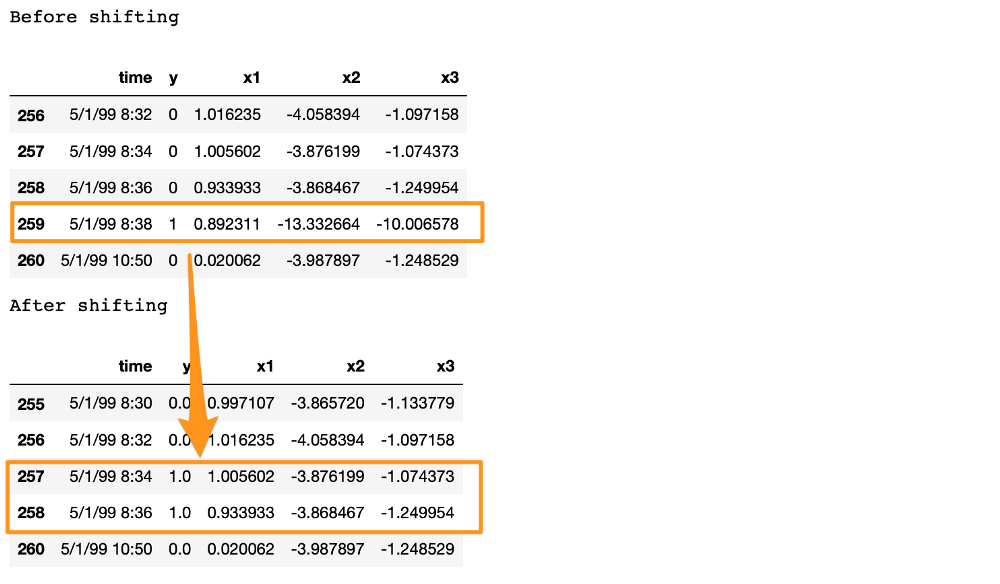如果我们在这里注意，我们将正面标签在5/1/99 8:38移动到n-1和n-2个时间戳，并且删除了行n。 此外，中断行和下一行之间的时间差超过2分钟。 这是因为，当发生中断时，机器会暂停状态一段时间。 在此期间，连续行的y = 1。 在提供的数据中，删除这些连续的中断行以防止分类器在已经发生之后学习预测中断。 有关详细信息，请参阅。
在继续之前，我们通过删除时间和其他两个分类列来清理数据。
# Remove time column, and the categorical columns
df = df.drop(['time', 'x28', 'x61'], axis=1)

Prepare Input Data for LSTM
LSTM比其他型号要求更高。 准备适合LSTM的数据可能需要大量的时间和精力。 但是，这通常是值得的。
LSTM模型的输入数据是三维数组。 数组的形状是样本x回溯x特征。 让我们理解他们，
samples：这只是观察的数量，换句话说，就是数据点的数量。 回顾：LSTM模型旨在回顾过去。 意思是，在时间t，LSTM将处理数据直到（t-lookback）进行预测。 特征：它是输入数据中存在的特征数。 首先，我们将提取功能和响应。
input_X = df.loc[:, df.columns != 'y'].values  # converts the df to a numpy array
input_y = df['y'].values

n_features = input_X.shape  # number of features

这里的input_X是大小样本x特征的二维数组。 我们希望能够将这样的2D数组转换为大小的3D数组：samples x lookback x features。 请参阅上面的图1以获得视觉理解。
为此，我们开发了一个函数temporalize。
def temporalize(X, y, lookback):
X = []
y = []
for i in range(len(input_X)-lookback-1):
t = []
for j in range(1,lookback+1):
# Gather past records upto the lookback period
t.append(input_X[[(i+j+1)], :])
X.append(t)
y.append(input_y[i+lookback+1])
return X, y

为了测试和演示此函数，我们将使用lookback = 5查看下面的示例。
print('First instance of y = 1 in the original data')
display(df.iloc[(np.where(np.array(input_y) == 1)-5):(np.where(np.array(input_y) == 1)+1), ])
lookback = 5  # Equivalent to 10 min of past data.
# Temporalize the data
X, y = temporalize(X = input_X, y = input_y, lookback = lookback)
print('For the same instance of y = 1, we are keeping past 5 samples in the 3D predictor array, X.')
display(pd.DataFrame(np.concatenate(X[np.where(np.array(y) == 1)], axis=0 )))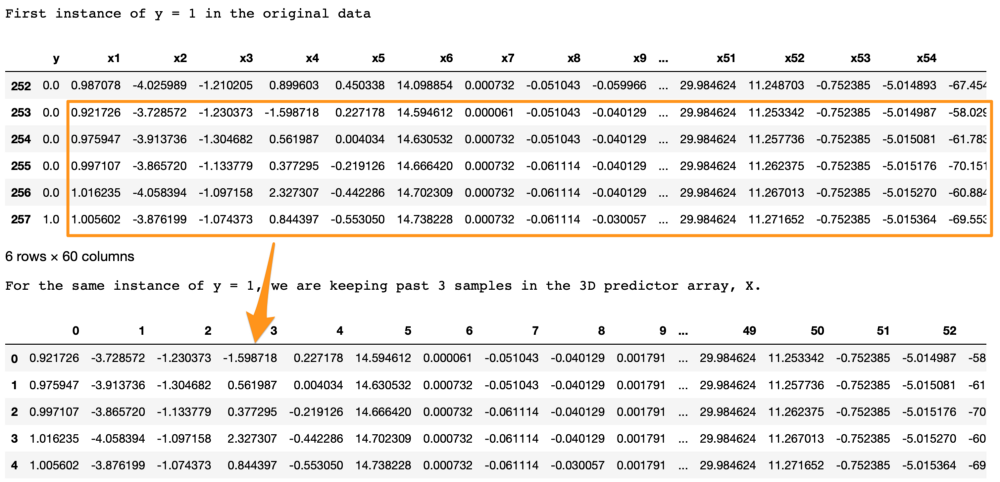我们在这里寻找的是，
在原始数据中，第257行y = 1。 使用lookback = 5，我们希望LSTM查看第257行（包括其自身）之前的5行。 在3D阵列X中，X [i，：，]处的每个2D块表示对应于y [i]的预测数据。 为了得出类比，在回归中y [i]对应于1D向量X [i，：]; 在LSTM中，y [i]对应于2D阵列X [i，：，]。 这个2D块X [i，：，]应该在input_X [i，：]处具有预测变量，并且前面的行应该具有给定的回溯。 正如我们在上面的输出中所看到的，底部的X [i，：，]块与顶部显示的y = 1的前五行相同。 同样，这适用于所有y的整个数据。 此处的示例显示为y = 1的实例，以便于可视化。### Split into train, valid, and test This is straightforward with the sklearn function.
X_train, X_test, y_train, y_test = train_test_split(np.array(X), np.array(y), test_size=DATA_SPLIT_PCT, random_state=SEED)
X_train, X_valid, y_train, y_valid = train_test_split(X_train, y_train, test_size=DATA_SPLIT_PCT, random_state=SEED)

为了训练自动编码器，我们将使用仅来自负标记数据的X. 因此，我们将对应于y = 0的X分开。
X_train_y0 = X_train[y_train==0]
X_train_y1 = X_train[y_train==1]
X_valid_y0 = X_valid[y_valid==0]
X_valid_y1 = X_valid[y_valid==1]

我们将把X重塑为所需的3D维度：样本x回溯x特征。
X_train = X_train.reshape(X_train.shape, lookback, n_features)
X_train_y0 = X_train_y0.reshape(X_train_y0.shape, lookback, n_features)
X_train_y1 = X_train_y1.reshape(X_train_y1.shape, lookback, n_features)
X_valid = X_valid.reshape(X_valid.shape, lookback, n_features)
X_valid_y0 = X_valid_y0.reshape(X_valid_y0.shape, lookback, n_features)
X_valid_y1 = X_valid_y1.reshape(X_valid_y1.shape, lookback, n_features)
X_test = X_test.reshape(X_test.shape, lookback, n_features)

Standardize the Data
通常最好使用标准化数据（转换为高斯，平均值为0，标准差为1）用于自动编码器。
一个常见的标准化错误是：我们将整个数据标准化，然后分成列车测试。 这是不正确的。 在建模过程中，测试数据应该完全看不到任何东西。 因此，我们应该对训练数据进行标准化，并使用其摘要统计数据来规范化测试数据（对于标准化，这些统计数据是每个特征的均值和方差）。 标准化这些数据有点棘手。 这是因为X矩阵是3D，我们希望标准化相对于原始2D数据发生。
为此，我们将需要两个UDF。
flatten：此函数将重新创建从中创建3D阵列的原始2D阵列。 该函数是temporalize的倒数，意味着X = flatten（temporalize（X））。 scale：此函数将缩放我们创建的3D数组作为LSTM的输入。
def flatten(X):
'''
Flatten a 3D array.

Input
X            A 3D array for lstm, where the array is sample x timesteps x features.

Output
flattened_X  A 2D array, sample x features.
'''
flattened_X = np.empty((X.shape, X.shape))  # sample x features array.
for i in range(X.shape):
flattened_X[i] = X[i, (X.shape-1), :]
return(flattened_X)

def scale(X, scaler):
'''
Scale 3D array.

Inputs
X            A 3D array for lstm, where the array is sample x timesteps x features.
scaler       A scaler object, e.g., sklearn.preprocessing.StandardScaler, sklearn.preprocessing.normalize

Output
X            Scaled 3D array.
'''
for i in range(X.shape):
X[i, :, :] = scaler.transform(X[i, :, :])

return X

为什么我们不首先规范原始2D数据然后创建3D数组？ 因为，要做到这一点，我们将：将数据拆分为训练和测试，然后进行标准化。 但是，当我们在测试数据上创建3D数组时，我们会丢失最初的样本行直到回顾。 拆分为train-valid-test将导致验证和测试集。 我们将从sklearn中安装标准化对象。 此功能将数据标准化为Normal（0,1）。 请注意，我们需要展平X_train_y0数组以传递给fit函数。
# Initialize a scaler using the training data.
scaler = StandardScaler().fit(flatten(X_train_y0))

We will use our UDF, scale, to standardize X_train_y0 with the fitted transform object scaler.
X_train_y0_scaled = scale(X_train_y0, scaler)

Make sure the scale worked correctly?
X_train的正确转换将确保扁平X_train的每列的均值和方差分别为0和1。 我们测试一下。
a = flatten(X_train_y0_scaled)
print('colwise mean', np.mean(a, axis=0).round(6))
print('colwise variance', np.var(a, axis=0))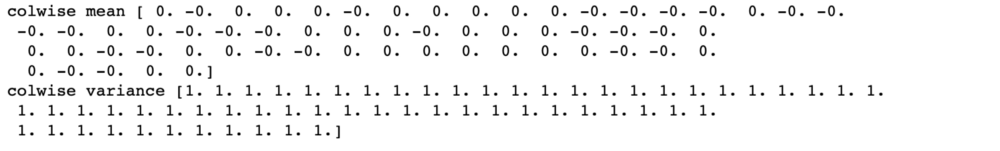上面输出的所有均值和方差分别为0和1。 因此，缩放是正确的。 我们现在将扩展验证和测试集。 我们将再次在这些集合上使用缩放器对象。
X_valid_scaled = scale(X_valid, scaler)
X_valid_y0_scaled = scale(X_valid_y0, scaler)
X_test_scaled = scale(X_test, scaler)

LSTM Autoencoder Training
We will, first, initialize few variables.
timesteps =  X_train_y0_scaled.shape # equal to the lookback
n_features =  X_train_y0_scaled.shape # 59, the number of variables

epochs = 500
batch = 64
lr = 0.0001

We, now, develop a simple architecture.
lstm_autoencoder = Sequential()
# Encoder
# Decoder

lstm_autoencoder.summary()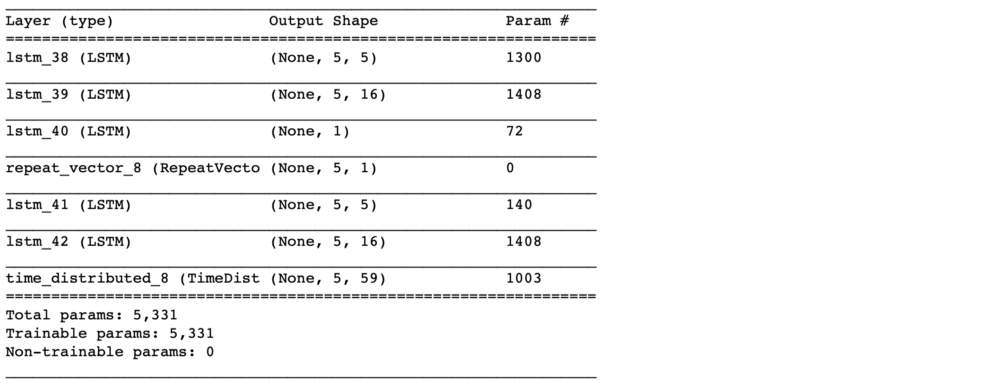从summary（）中，参数总数为5,331。 这大约是培训规模的一半。 因此，这是一个合适的模型。 要拥有更大的架构，我们需要添加正则化，例如 辍学，将在下一篇文章中介绍。
现在，我们将训练自动编码器。
adam = optimizers.Adam(lr)

cp = ModelCheckpoint(filepath="lstm_autoencoder_classifier.h5",
save_best_only=True,
verbose=0)

tb = TensorBoard(log_dir='./logs',
histogram_freq=0,
write_graph=True,
write_images=True)

lstm_autoencoder_history = lstm_autoencoder.fit(X_train_y0_scaled, X_train_y0_scaled,
epochs=epochs,
batch_size=batch,
validation_data=(X_valid_y0_scaled, X_valid_y0_scaled),
verbose=2).history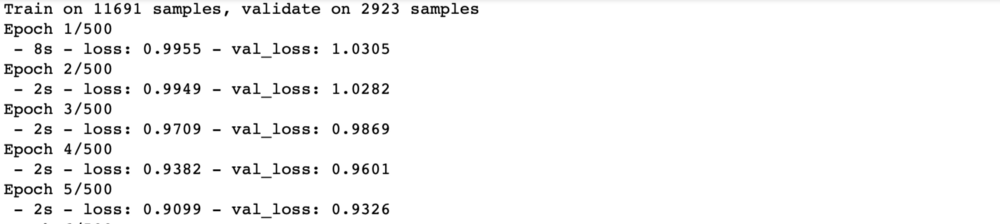Plotting the change in the loss over the epochs.
plt.plot(lstm_autoencoder_history['loss'], linewidth=2, label='Train')
plt.plot(lstm_autoencoder_history['val_loss'], linewidth=2, label='Valid')
plt.legend(loc='upper right')
plt.title('Model loss')
plt.ylabel('Loss')
plt.xlabel('Epoch')
plt.show()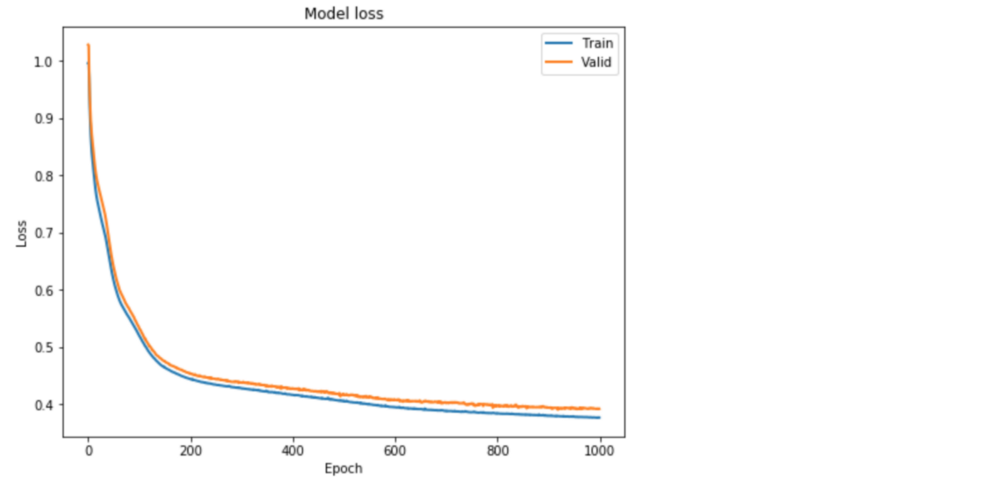Classification
Similar to the previous post , 这里我们展示了如何使用自动编码器重建错误进行稀有事件分类。 我们遵循这个概念：自动编码器有望重建一个重建错误很高的noif，我们将其归类为一个分页符。
我们需要确定这个门槛。 另请注意，这里我们将使用包含y = 0或1的整个验证集。
valid_x_predictions = lstm_autoencoder.predict(X_valid_scaled)
mse = np.mean(np.power(flatten(X_valid_scaled) - flatten(valid_x_predictions), 2), axis=1)
error_df = pd.DataFrame({'Reconstruction_error': mse,
'True_class': y_valid.tolist()})
precision_rt, recall_rt, threshold_rt = precision_recall_curve(error_df.True_class, error_df.Reconstruction_error)
plt.plot(threshold_rt, precision_rt[1:], label="Precision",linewidth=5)
plt.plot(threshold_rt, recall_rt[1:], label="Recall",linewidth=5)
plt.title('Precision and recall for different threshold values')
plt.xlabel('Threshold')
plt.ylabel('Precision/Recall')
plt.legend()
plt.show()

Note that we have to flatten the arrays to compute the mse.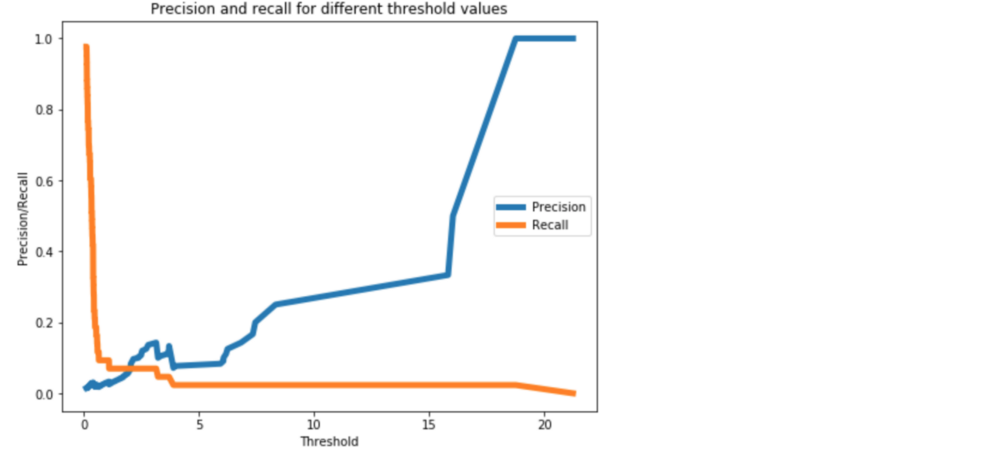图3. 0.8的阈值应该在精确度和召回率之间提供合理的权衡，因为我们希望更高的召回率。 现在，我们将对测试数据进行分类。
我们不应该从测试数据估计分类阈值。 这会导致过度拟合。
threshold_fixed = 0.7
groups = error_df.groupby('True_class')
fig, ax = plt.subplots()
for name, group in groups:
ax.plot(group.index, group.Reconstruction_error, marker='o', ms=3.5, linestyle='',
label= "Fraud" if name == 1 else "Normal")
ax.hlines(threshold_fixed, ax.get_xlim(), ax.get_xlim(), colors="r", zorder=100, label='Threshold')
ax.legend()
plt.title("Reconstruction error for different classes")
plt.ylabel("Reconstruction error")
plt.xlabel("Data point index")
plt.show();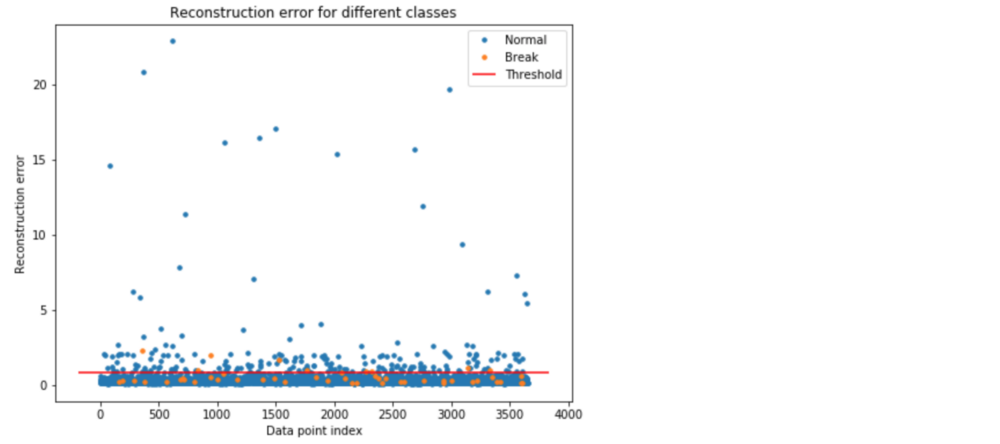图4.使用阈值= 0.8进行分类。 阈值线上方的橙色和蓝色点分别代表真阳性和假阳性。 在图4中，阈值线上方的橙色和蓝色点分别代表真阳性和假阳性。 我们可以看到，我们有很多误报。
让我们看看准确性结果。
Test Accuracy
Confusion Matrix
pred_y = [1 if e > threshold_fixed else 0 for e in error_df.Reconstruction_error.values]
conf_matrix = confusion_matrix(error_df.True_class, pred_y)
plt.figure(figsize=(12, 12))
sns.heatmap(conf_matrix, xticklabels=LABELS, yticklabels=LABELS, annot=True, fmt="d");
plt.title("Confusion matrix")
plt.ylabel('True class')
plt.xlabel('Predicted class')
plt.show()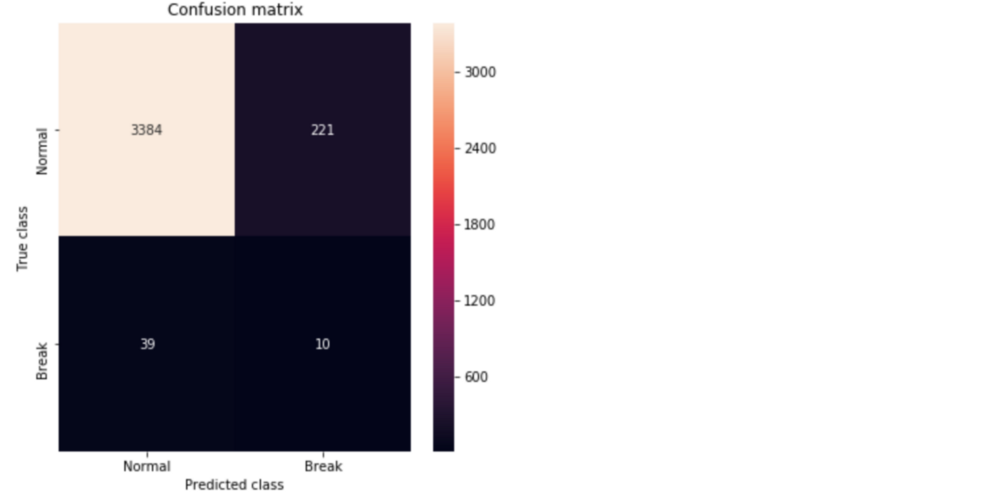Figure 5. Confusion matrix showing the True Positives and False Positives.
ROC Curve and AUC
false_pos_rate, true_pos_rate, thresholds = roc_curve(error_df.True_class, error_df.Reconstruction_error)
roc_auc = auc(false_pos_rate, true_pos_rate,)
plt.plot(false_pos_rate, true_pos_rate, linewidth=5, label='AUC = %0.3f'% roc_auc)
plt.plot([0,1],[0,1], linewidth=5)
plt.xlim([-0.01, 1])
plt.ylim([0, 1.01])
plt.legend(loc='lower right')
plt.ylabel('True Positive Rate')
plt.xlabel('False Positive Rate')
plt.show()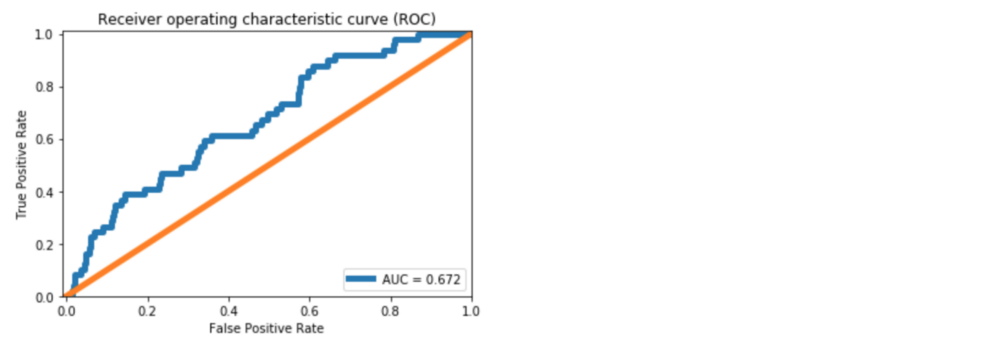图6. ROC曲线。 与中的密集层自动编码器相比，我们看到AUC提高了约10％。 从图5中的Confusion Matrix，我们可以预测39个中断实例中的10个。 正如中所讨论的，这对造纸厂来说意义重大。 然而，与密集层自动编码器相比，我们实现的改进很小。
主要原因是LSTM模型有更多的参数来估算。 使用LSTM正则化变得很重要。 正规化和其他模型改进将在下一篇文章中讨论。
Github repository
What can be done better?
In the next article, we will learn tuning an Autoencoder. We will go over,
CNN LSTM Autoencoder, Dropout layer, LSTM Dropout (Dropout_U and Dropout_W) Gaussian-dropout layer SELU activation, and alpha-dropout with SELU activation.
Conclusion
这篇文章继续在中关于极端罕见事件二进制标记数据的工作。 为了利用时间模式，LSTM Autoencoders用于为多变量时间序列过程构建罕见的事件分类器。 讨论了有关LSTM模型的数据预处理步骤的详细信息。 训练简单的LSTM自动编码器模型并用于分类。 发现了对密集自动编码器的准确性的一些改进。 为了进一步改进，我们将在下一篇文章中探讨使用Dropout和其他技术改进Autoencoder的方法。
References
[Extreme Rare Event Classification using Autoencoders in Keras](https://towardsdatascience.com/extreme-rare-event-classification-using-autoencoders-in-keras-a565b386f0980Ranjan, C., Mustonen, M., Paynabar, K., & Pourak, K. (2018). Dataset: Rare Event Classification in Multivariate Time Series. arXiv preprint arXiv:1809.10717Time-series forecasting with deep learning & LSTM autoencodersComplete code: LSTM Autoencoder












展开全文• 堆栈式自编码器的原理请看： https://blog.csdn.net/hjimce/article/details/49106869 这里直接进行代码的实现 代码结构分为：1.mnist的读取，2.数据预处理，3.模型的训练过程。 1.mnist的读取 ''' 采用keras的堆栈...
堆栈式自编码器的原理请看： https://blog.csdn.net/hjimce/article/details/49106869 这里直接进行代码的实现 代码结构分为：1.mnist的读取，2.数据预处理，3.模型的训练过程。
1.mnist的读取
''' 采用keras的堆栈式Autoencode 将mnist的图片进行分类'''
import numpy as np
import os
import struct
np.random.seed(2018)

from keras.datasets import mnist
from keras.models import Model
from keras.layers import Dense,Input
from keras.utils.np_utils import to_categorical
import matplotlib.pyplot as plt

# 训练集 shape (60,000,28*28) 测试集 shape (10000,)
''' load MNIST data from path'''
labels_path=os.path.join(path,'%s-labels.idx1-ubyte' % kind)
images_path=os.path.join(path,'%s-images.idx3-ubyte' % kind)

with open(labels_path,'rb') as lbpath:
labels=np.fromfile(lbpath,dtype=np.uint8)
with open(images_path,'rb') as impath:
images=np.fromfile(impath,dtype=np.uint8).reshape(len(labels),784)
return images,labels

y_train_cate= to_categorical(y_train, num_classes=10)
y_test_cate= to_categorical(y_test, num_classes=10)

# 显示mnist图片
# 图形2*5 灰度值
fig,ax=plt.subplots(nrows=2,ncols=5,sharex=True,sharey=True)
ax=ax.flatten()
for i in range(10):
img=X_train[y_train==i].reshape(28,28)
ax[i].imshow(img,cmap='Greys',interpolation='nearest')

ax.set_xticks([])
ax.set_yticks([])
plt.tight_layout()
plt.show()


当然也可以直接采用keras中的函数 mnist.load_data()获取数据，但是由于数据量比较大，有可能直接下载不成功，所以我们直接采用将数据下载到本地文件夹中，然后再进行读取。数据下载链接：http://yann.lecun.com/exdb/mnist/ 在数据读取过程中，需要特别注意的地方数据格式是idx1-ubyte，idx3-ubyte的格式，需要进行转换。 详细解释代码： load_mnist 函数返回两个数组, 第一个是一个 n x m 维的 NumPy array(images), 这里的 n 是样本数(行数), m 是特征数(列数). 训练数据集包含 60,000 个样本, 测试数据集包含 10,000 样本. 在 MNIST 数据集中的每张图片由 28 x 28 个像素点构成, 每个像素点用一个灰度值表示. 在这里, 我们将 28 x 28 的像素展开为一个一维的行向量, 这些行向量就是图片数组里的行(每行 784 个值, 或者说每行就是代表了一张图片). load_mnist 函数返回的第二个数组(labels) 包含了相应的目标变量, 也就是手写数字的类标签(整数 0-9). 为了理解这两行代码, 我们先来看一下 MNIST 网站上对数据集的介绍:
TRAINING SET LABEL FILE (train-labels-idx1-ubyte):

[offset] [type]          [value]          [description]
0000     32 bit integer  0x00000801(2049) magic number (MSB first)
0004     32 bit integer  60000            number of items
0008     unsigned byte   ??               label
0009     unsigned byte   ??               label
........
xxxx     unsigned byte   ??               label
The labels values are 0 to 9.

通过使用上面两行代码, 我们首先读入 magic number, 它是一个文件协议的描述, 也是在我们调用 fromfile 方法将字节读入 NumPy array 之前在文件缓冲中的 item 数(n). 作为参数值传入 struct.unpack 的 >II 有两个部分:

: 这是指大端(用来定义字节是如何存储的); 如果你还不知道什么是大端和小端, Endianness 是一个非常好的解释. (关于大小端, 更多内容可见<<深入理解计算机系统 – 2.1 节信息存储>>) I: 这是指一个无符号整数. 通过执行下面的代码, 我们将会从刚刚解压 MNIST 数据集后的 mnist 目录下加载 60,000 个训练样本和 10,000 个测试样本.

为了了解 MNIST 中的图片看起来到底是个啥, 让我们来对它们进行可视化处理. 从 feature matrix 中将 784-像素值 的向量 reshape 为之前的 28*28 的形状, 然后通过 matplotlib 的 imshow 函数进行绘制。
2.数据预处理
# 数据预处理
X_train=X_train.astype('float32')/255-0.5 # minmax_normalized(归一化在（-0.5,0.5）)之间
X_test=X_test.astype('float32')/255-0.5 # minmax_normalized
X_train_len=X_train.shape
X_test_len=X_test.shape

X_train=X_train.reshape((X_train_len,-1))
X_test=X_test.reshape((X_test_len,-1))

print(X_train.shape,X_test.shape)

主要是将灰度图像值进行（-0.5,0。5）的归一化
3.模型训练
自编码网路结构采用（784，128,64,10,10,64，128,684）的结构进行无监督训练。
nput_img=Input(shape=(784,))
# 编码层
encoded=Dense(128,activation='relu',name='encoded_hidden1')(input_img)
encoder_output=Dense(64,activation='relu',name='encoded_hidden2')(encoded)
LR=Dense(10,activation='softmax',name='LR')(encoder_output)

# 解码层
decoded=Dense(64,activation='relu',name='decoded_hidden2')(encoder_output)
decoded=Dense(128,activation='relu',name='decoded_hidden3')(decoded)
decoded=Dense(784,activation='tanh',name='decoded_output')(decoded)

# 构建自编码模型
autoencoder=Model(inputs=input_img,outputs=decoded)

# complile autoencoder 设置自编码的优化参数
# train
hist=autoencoder.fit(X_train,X_train,epochs=20,batch_size=250,shuffle=True)

中间设置一个LR层，进行后面的多分类输出层。 下面是多分类的模型训练过程：
采用编码层的网络结构，从新构成一个新的model，此model的参数跟原来autoencode的训练的参数一样。
encoder=Model(inputs=input_img,outputs=LR)
encoder.fit(X_train,y_train_cate,epochs=20,batch_size=250,shuffle=True)
core=encoder.evaluate(X_test,y_test_cate)
print(score)
print(encoder.summary())

评估结果与网络参数如下：
score:
[0.09663939199754969, 0.9704]
_________________________________________________________________
Layer (type)                 Output Shape              Param #
=================================================================
input_1 (InputLayer)         (None, 784)               0
_________________________________________________________________
encoded_hidden1 (Dense)      (None, 128)               100480
_________________________________________________________________
encoded_hidden2 (Dense)      (None, 64)                8256
_________________________________________________________________
LR (Dense)                   (None, 10)                650
=================================================================
Total params: 109,386
Trainable params: 109,386
Non-trainable params: 0

可以看出准确率与loss都很好。 参考链接： http://yann.lecun.com/exdb/mnist/ https://www.cnblogs.com/wzdLY/p/9683657.html https://blog.csdn.net/simple_the_best/article/details/75267863 https://blog.csdn.net/hjimce/article/details/49106869
展开全文keras mnist SAE
• MINDFUL是一种深度学习模型，通过结合自动编码器和一维卷积神经网络来执行二进制分类。 class MindfulNET.MINDFUL.MIDFUL_NET(dsConfig, autoencoderA=None,autoencoderN=None,model=None) 参数： dsConfig ：参数...Python
• 【翻译自： Autoencoder Feature Extraction for Classification】 【说明：Jason BrownleePhD大神...编码器压缩输入，而解码器尝试根据编码器提供的压缩版本重新创建输入。训练后，将保存编码器模型，并丢弃解码...
• ## Pytorch实现自编码器

千次阅读 2020-02-26 09:08:22
什么是编码器 有一中数据压缩的、降维的意思 举个例子来说明，同一张图片，高清的和标清的我们都能识别出图片中的内容（这里就考虑识别这一个需求，其他需求暂不考虑），这是因为即使是标清的图片，也保留了进行...pytorch 深度学习 神经网络
• 结合自编码器技术和改进的K-means聚类技术针对网络流量实现了未知协议的分类识别。利用自编码器对网络流量进行降维和特征提取，使用聚类技术对降维后数据进行无监督的分类，最终实现对网络流量的无监督识别分类。...特征提取
• 本文转载自《机器之心》，原文链接：...Mobibit 创始人兼 CEO Pramod Chandrayan 近日在 codeburst.io 上发文对自动编码器的基础知识和类型进行了介绍并给出了代码实例。机器之心对本文进行了...深度学习
• 编码器是一种特殊的神经网络（neural network），它的输出目标（target）就是输入（所以它基本上就是试图将输出重构为输入），由于它不需要任何人工标注，所以可以采用无监督的方式进行训练。 自编码器包括两...深度学习 MNIST
• 针对智能电网日益突出的电能质量扰动问题，提出了一种基于稀疏自动编码器(SAE)深度神经网络的电能质量扰动分类方法。利用SAE对电能质量扰动原始数据进行无监督特征学习，自动提取数据特征的稀疏特征表达；通过堆栈式...
• 基于这些缺陷，在这项工作中，我们认为这些任务是相对的，并使用权重共享参数来学习神经网络模型中文本的表示形式，我们引入并研究了一种具有变分自动编码器生成模型的多任务方法（ MTVAE）通过共同学习它们。...
• 行业分类-物理装置-磁编码器模块、磁编码器以及伺服电机.zip
• 文章目录一、自编码器（Autoencoder, AE）自编码器的结构和思想结构思想自编码器的作用与类型作用类型二、Tensorflower代码实现普通自编码器多层自编码器卷积自编码器稀疏自编码器 一、自编码器（Autoencoder, AE） ...
• 本文主要讲了编码器工作原理分类，下面一起来学习一下
•研究论文
• 为降低人工成本,提出了一种基于稀疏自编码器的烟叶成熟度分类算法,从计算机视觉角度自动识别烟叶的成熟度。首先对烟叶数字图像进行去除背景、归一化等预处理操作;其次从无监督学习算法入手,利用稀疏自编码器构建特征...
• 行业分类-电子电器-编码器安装结构及电机.zip
• 行业分类-电器装置-一种磁性编码器及电机.zip...